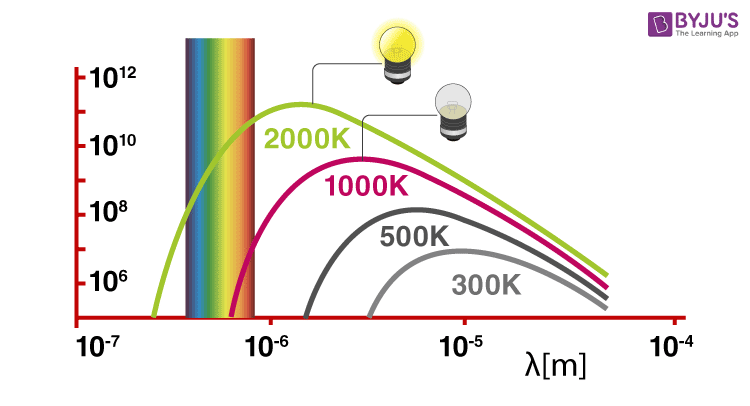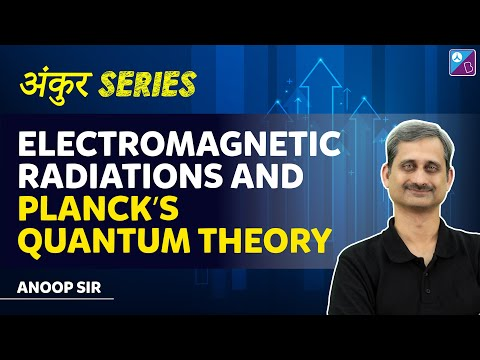# Planck's Quantum Theory - Quantization Of Energy

## Introduction

Before learning about Planck’s quantum theory, we need to know a few things.

As progress in the science field was happening, Maxwell’s suggestion about the wave nature of electromagnetic radiation was helpful in explaining phenomena such as interference, diffraction, etc. However, he failed to explain various other observations such as the nature of emission of radiation from hot bodies, photoelectric effect, i.e. ejection of electrons from a metal compound when electromagnetic radiation strikes it, the dependence of heat capacity of solids upon temperature, line spectra of atoms (especially hydrogen).

Solids, when heated, emit radiation varying over a wide range of wavelengths. For example: when we heat solid colour, changes continue with a further increase in temperature. This change in colour happens from a lower frequency region to a higher frequency region as the temperature increases. For example, in many cases, it changes from red to blue. An ideal body which can emit and absorb radiation of all frequencies is called a black body. The radiation emitted by such bodies is called black body radiation.

Thus, we can say that variation of frequency for black body radiation depends on the temperature. At a given temperature, the intensity of radiation is found to increase with an increase in the wavelength of radiation which increases to a maximum value and then decreases with an increase in the wavelength. This phenomenon couldn’t be explained with the help of Maxwell’s suggestions. Hence, Planck proposed Planck’s quantum theory to explain this phenomenon.

## Recommended Videos## Planck’s Quantum Theory

According to Planck’s quantum theory,

1. Different atoms and molecules can emit or absorb energy in discrete quantities only. The smallest amount of energy that can be emitted or absorbed in the form of electromagnetic radiation is known as quantum.
2. The energy of the radiation absorbed or emitted is directly proportional to the frequency of the radiation.Meanwhile, the energy of radiation is expressed in terms of frequency as,

E = h ν

Where,

E = Energy of the radiation

h = Planck’s constant (6.626×10–34 J.s)

Interestingly, Planck has also concluded that these were only an aspect of the processes of absorption and emission of radiation. They had nothing to do with the physical reality of the radiation itself. Later in the year 1905, famous German physicist, Albert Einstein also reinterpreted Planck’s theory to further explain the photoelectric effect. He was of the opinion that if some source of light was focused on certain materials, they can eject electrons from the material. Basically, Planck’s work led Einstein in determining that light exists in discrete quanta of energy, or photons.

## Electromagnetic Radiations & Planck’s Quantum TheoryQ1

### What is a Planck curve?

A black body’s energy density between λ and λ + dλ is the energy of a mode E = hc / λ times the density of photon states, times the probability that the mode is filled. This is the famous formula from Planck for a black body’s energy density.

Q2

### What is Stefan’s law of radiation?

The law of Stefan-Boltzmann states that the overall radiant heat power released from a surface is proportional to its fourth absolute temperature power. The rule only refers to black bodies, imaginary surfaces that collect heat radiation from all events.

Q3

### How is blackbody radiation produced?

Electromagnetic radiation is produced from all objects according to their temperature. An idealised object that consumes the electromagnetic energy that it comes into contact with is a black body. In a continuous continuum, which then emits thermal radiation according to its temperature.

Q4

### What is Planck’s constant in simple terms?

The Planck constant compares the sum of energy a photon bears with its electromagnetic wave frequency. It is named after Max Planck, the physicist. In quantum mechanics, it is an essential quantity.

Q5

### What is Planck’s number?

Planck’s constant is currently calculated by scientists to be 6.62607015 x 10-34 joule-seconds. In 1900, Planck identified his game-changing constant by describing how the smallest bits of matter release energy in discrete bundles called quanta, essentially placing the “quanta” in quantum mechanics.

Test your knowledge on planks quantum theory

1. i liked your material you have given

2. Thank you so much.

3. I like it. It helps with my chemistry homework.

4. Good job keep it.

5. It’s good

6. softly captured, keep it up

7. thanks

8. thnx a lott

9. love you BYJU’S
FOREVER

10. thank you so much, helped with my essay on Max Planck

11. Thank you byjus awesome it helped me a lot in my project